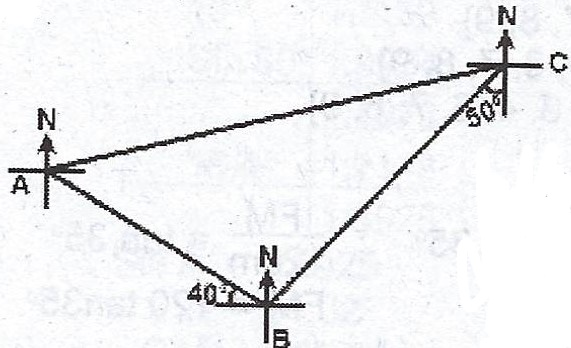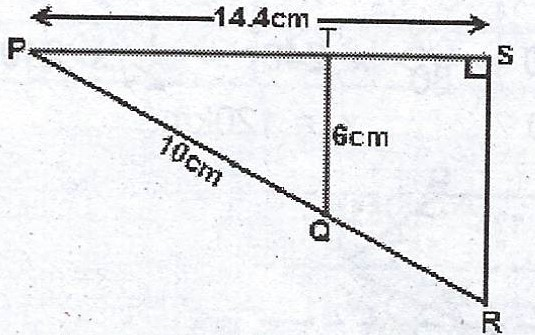### Mathematics Past Questions

3731

Using ruler and a pair of compasses only,

(a) construct (i) a quadrilateral PQRS with /PS/ = 6 cm, < RSP = 9 cm, /QR/ = 8.4 cm and /PQ/ = 5.4 cm;   (ii) the bisectors of < RSP and < SPQ to meet at X ;                         (iii) the perpendicular XT to meet PS at T.

(b) Measure /XT/.

3732In the diagram, /AB/ = 8 km, /BC/ = 13 km, the bearing of A from B is 310° and the bearing of B from C is 230°. Calculate, correct to 3 significant figures,

(a) the distance AC ;

(b) the bearing of C from A ;

(c) how far east of B, C is.

3733

(a) Copy and complete the table of values for the relation $$y = -x^{2} + x + 2; -3 \leq x \leq 3$$.

 x -3 -2 -1 0 1 2 3 y -4 2 -4

(b) Using scales of 2 cm to 1 unit on the x- axis and 2 cm to 2 units on the y- axis, draw a graph of the relation $$y = -x^{2} + x + 2$$.

(c) From the graph, find the : (i) minimum value of y ; (ii) roots of equation $$x^{2} - x - 2 = 0$$ ; (iii) gradient of the curve at x = -0.5.

3734

(a)In the diagram, < PTQ = < PSR = 90°, /PQ/ = 10 cm, /PS/ = 14.4 cm and /TQ/ = 6 cm. Calculate the area of the quadrilateral QRST.

(b) Two opposite sides of a square are each decreased by 10% while the other two are each increased by 15% to form a rectangle. Find the ratio of the area of the rectangle to that of the square.

3735

The frequency distribution of the weight of 100 participants in a high jump competition is as shown below :

 Weight (kg) 20 - 29 30 - 39 40 - 49 50 - 59 60 - 69 70 - 79 Number of  Participants 10 18 22 25 16 9

(a) Construct the cumulative frequency table.

(b) Draw the cumulative frequency curve.

(c) From the curve, estimate the : (i) median ; (ii) semi- interquartile range ; (iii) probability that a participant chosen at random weighs at least 60 kg.